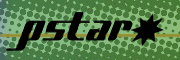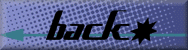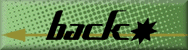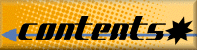### 3. PSTAR and ASTAR Databases for Protons and Helium Ions

Access the PSTAR or ASTAR Databases

3.1. Overview

There are two ways to use the PSTAR and ASTAR databases. The full-featured version gives the user many capabilities (i.e., file-uploading, graphing, and formatted tables). The text-based version only outputs a basic text table of data. This may be useful if the data are to be exported to the user's speadsheet program.

3.2. Output of PSTAR and ASTAR

The following quantities are calculated:

 a Electronic (collision) stopping power, MeV cm2/g; b Nuclear stopping power, MeV cm2/g; c Total stopping power (sum of a and b), MeV cm2/g; d CSDA (continuous-slowing-down approximation) range, g/cm2; e Projected range, g/cm2; f Detour factor (ratio of projected range to CSDA range).

The significance of these quantities is indicated in the Appendix.

The graphical version of the database also has the abilty to graph the calculated data. Next to the image are buttons that allow you to resize the image which may be useful if you are printing.

3.3. List of Materials

Table 2 lists the names of the 74 materials in the data files. The list includes 26 elements, and 48 compounds and mixtures.

The information used by PSTAR and ASTAR consists of:

 a The atomic numbers and fractions by weight of the constituent atoms; b The density at 20 °C, 101 325 Pa (1 atm); c The mean excitation energy of the material, which is a key parameter in Bethe's stopping power formula.

This compositional data may be examined (text-only [table-free] version).

3.4. List of Energies

PSTAR and ASTAR may be run with a list of over 100 default energies or with user defined energies. Note: Energies must not be smaller than 0.001 MeV, or greater than 10 000 MeV for protons or 1000 MeV for helium ions. Additional energies may be merged into the default list or the defaults can be excluded. There are two options for entering additional energies:

1) File uploading: (You must have a file upload compatible browser) Energy values should be entered in MeV and separated by  Return  or  Enter. The file should be in ASCII format containing one additional energy per line.

Example:

```0.01
1.23
2.03E2
5E-3
100.23
```

2) Manual input: A text box is provided for manual entry of additional energies. This is the only method available for browsers that do not handle file uploading. One should be able to copy and paste the energy values into this box. The format is one energy per line as above.

3.5. Methods Used To Evaluate Stopping Powers

Only a brief indication of the methods used for protons and helium ions is given here. For details, the reader is referred to the forthcoming ICRU Report 49 (ICRU, 1993). At high energies, collision stopping powers are evaluated using Bethe's stopping-power formula (Bethe, 1930). At low energies, fitting-formulas are used which are based on experimental stopping power data. The boundary between the high- and low-energy regions was at approximately 0.5 MeV for protons, and 2 MeV for alpha particles.

For each material, the experimental stopping powers at energies below a cut-off energy T1, together with the theoretical values from the Bethe theory at energies above a cut-off energy T2, are fitted by single cubic spline. Typical values are T1 = 0.2 MeV for protons and 1.0 MeV for helium ions, and T2 = 0.5 MeV for protons and 2.0 MeV for helium ions. This spline function is used for interpolation at energies between T1 and T2. The two cut-off energies are adjusted individually for each material to obtain a visually pleasing plot of stopping power vs. energy.

In ICRU (1993) the uncertainties of the collision stopping powers in the high-energy region are stated to be 1 % to 2 % for elements, and 1 % to 4 % for compounds. The uncertainties are more difficult to estimate in the low-energy region. In ICRU (1993) they are estimated to be 2 % to 5 % at 1000 keV, 5 % to 10 % at 100 keV, 10 % to 15 % at 10 keV, and at least 20 % to 30 % at 1 keV.

3.5.1. Stopping Power Theory at High Energies. The stopping power formula used in the high-energy region included various correction terms: (a) shell corrections, which are important when the proton velocity is not large compared to the velocities of the atomic electrons; (b) the Barkas and Bloch corrections, which take into account departures from the first-order Born approximation; and (c) the density-effect correction, which is noticeable only for protons with energies above several hundred MeV.

The values of the mean excitation energies used in the stopping power formula are the same as those adopted in ICRU Report 37 (ICRU, 1984). They are based on the analysis of measured stopping powers, and on information extracted from empirical oscillator strengths and dielectric response functions. For compounds, the mean excitation energies take into account, by a crude approximation, differences between the gaseous and condensed phase, and the effects of chemical binding.

The shell corrections for most elements are based on semi-empirical formulas developed by Bichsel and described in ICRU (1984). For elements with atomic numbers Z > 63, and for Z = 47, revised shell corrections from Bichsel (1991) are used. The Bloch correction is evaluated from the formula given by Bloch (1933). The Barkas correction is calculated according to the method of Ashley, Ritchie and Brandt (1972, 1973), with parameter values recommended by Bichsel (ICRU, 1984). For elements with atomic numbers Z ≥ 64, and for Z = 47, empirical formulas of Bichsel (1990) are used for the Barkas correction.

3.5.2. Empirical Stopping Powers at Low Energies. In the low-energy region, stopping powers are calculated from fitting formulas that represent experimental data for many elements and a limited number of compounds. Extensive use is made of a fitting formula of Varelas and Biersack (1970), with numerical coefficients adopted in ICRU (1993), which - except for a few materials - are from Andersen and Ziegler (1977) for protons, and from Ziegler (1977) or Watt (1988) for alpha particles. For elements without experimental data, stopping powers were obtained by Andersen and Ziegler (1977) and by Ziegler (1977) through interpolation with respect to atomic number, and were then fitted by the Varelas-Biersack formula. For a few materials a different fitting formula for alpha-particle stopping powers developed by Powers (1978) was used.

For compounds for which no experimental stopping power data are available, the Bragg additivity rule was used, and mass stopping powers were calculated as linear combinations of the mass stopping powers of the constituent atoms. When applying the additivity rule to mixtures, these are treated, to the extent possible, as a mixture of compounds and elements. For example, the stopping power of "muscle" is obtained as a weighted sum of the stopping power of water (assumed to be 78.6 % by weight) and the stopping powers of the other elemental constituents.

3.5.3. Nuclear Stopping Powers. Cross sections for the elastic scattering of charged particles by atoms are obtained by a classical-mechanics orbit calculation, using the method of Everhart, Stone and Carbone (1955). For protons the screened potential is assumed to be the Thomas-Fermi potential as parameterized by Molière (1947). For alpha particles, the "universal" ion-atom potential of Ziegler, Biersack and Littmark (1985) is used. Nuclear stopping powers are calculated using the relation between the deflection angles and the energy transfers to the recoiling atom in elastic collisions. In ICRU (1993) the uncertainties of nuclear stopping powers for alpha particles are estimated to be 5 % to 10 % at 100 keV, 10 % at 10 keV, and 10 % to 20 % at 1 keV.

3.5.4. CSDA and Projected Ranges. CSDA ranges are calculated in the continuous-slowing-down approximation by integrating the reciprocal of the total stopping power (collision plus nuclear) with respect to energy. Projected ranges are obtained in a transport calculation according to the method of Lewis (1950), which uses the elastic scattering cross sections discussed in Section 3.5.3.

Databasesto ASTAR             to PSTARText Versions

Introduction   |   ESTAR   |   PSTAR and ASTAR   |   References   |   Appendix